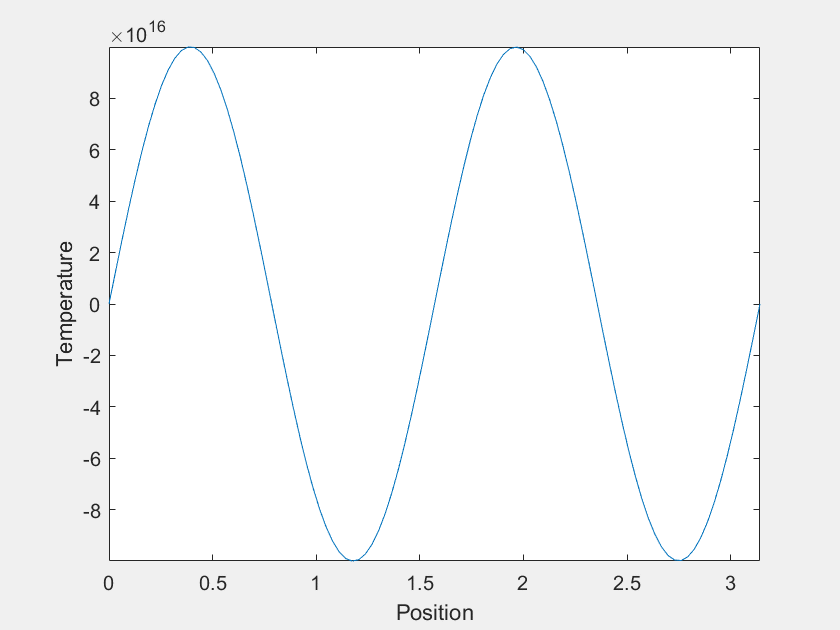# The Heat Equation

Here a special case of the 1D heat equation is considered. $$u_t(x,t)=u_{xx}(x,t)$$, with boundary conditions $$u(0,t)=0$$ and $$u(\pi,t)=0$$, and with initial condition $$u(x,0)=f(x)$$. The nuance will be in what we choose $$f(x)$$ to be.

The solution will be of the form:

$$u(x,t)=\Sigma_{n=1}^\infty a_n \sin (nx) e^{-n^2t}$$,

where $$a_n$$ are the fourier coefficients of $$f(x)$$.

Of course the $$n=1$$ mode becomes dominant at large $$t$$, but what about before that? For most $$f(x)$$, it is easy to imagine that $$u(x,t)$$ would just 'melt' until it becomes indistinguishable from the $$n=1$$ mode. However, consider the case where, for the first few terms, the value of each $$a_n$$ is much greater than the last. Each term decays much faster than the last as well, and one can imagine choosing the magnitudes of the $$a_n$$'s such that there is a period of time where each is dominant. This was done with the first four $$a_n$$ to yield the following:The temperature varies over more than 20 orders of magnitude, so maybe this isn't an observable phenomenon. The experimental setup I was imagining was a wire held in a vacuum with $$f(x)$$ imposed by lasers, but I suspect there is no material that is solid over a sufficient range of temperatures. Also, I am neglecting heat capacity's temperature dependence, which might complicate things.

The axes are distracting, so let's get rid of them. Also, this model can demonstrate extreme sensitivity to initial conditions. Neglecting $$a_2$$ and $$a_3$$ only changes $$f(x)$$ by about one part in a thousand, but it has a dramatic outcome on the systems trajectory.

With $$a_2$$ and $$a_3$$ Without $$a_2$$ and $$a_3$$Last Updated: 5/21/2020